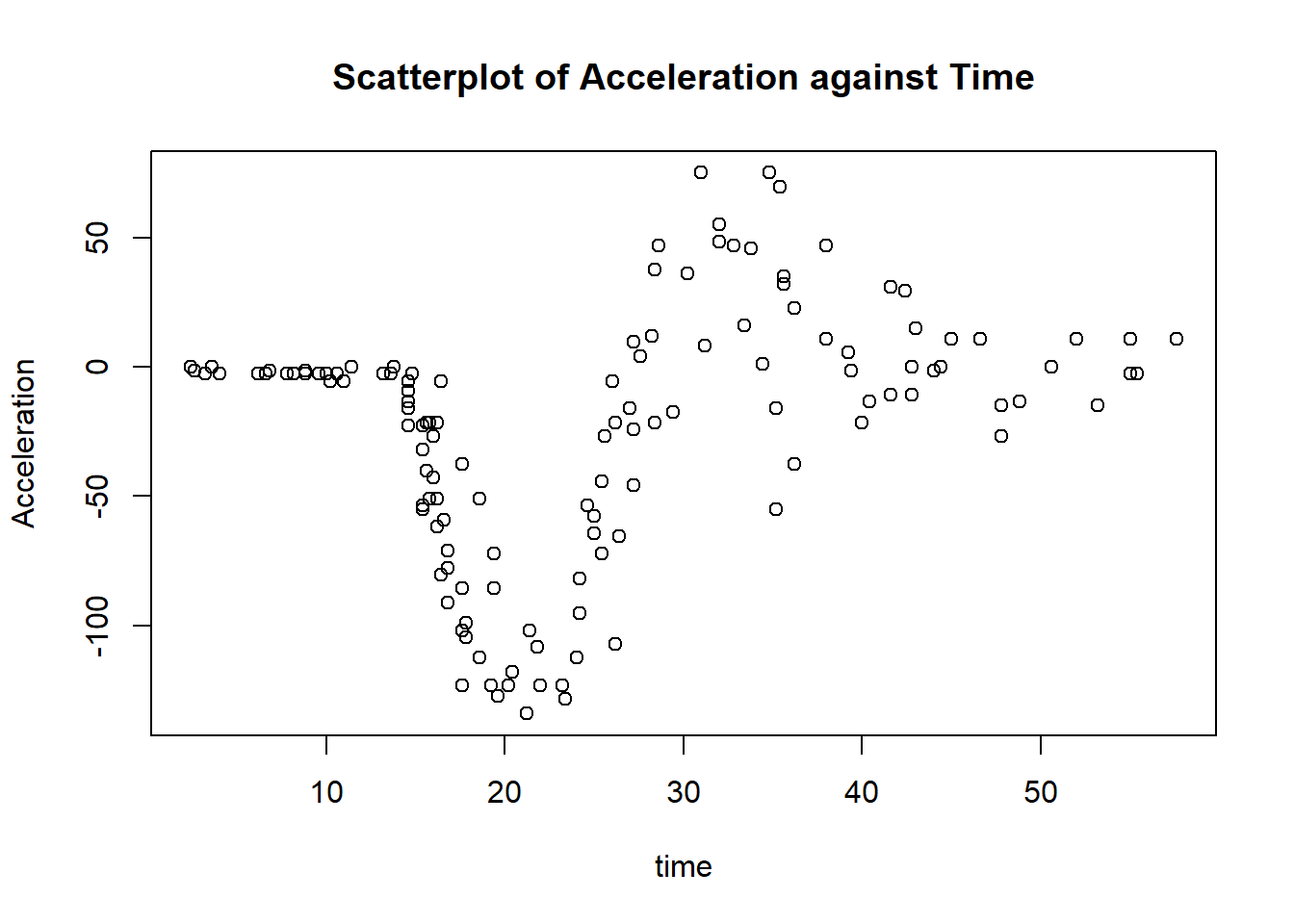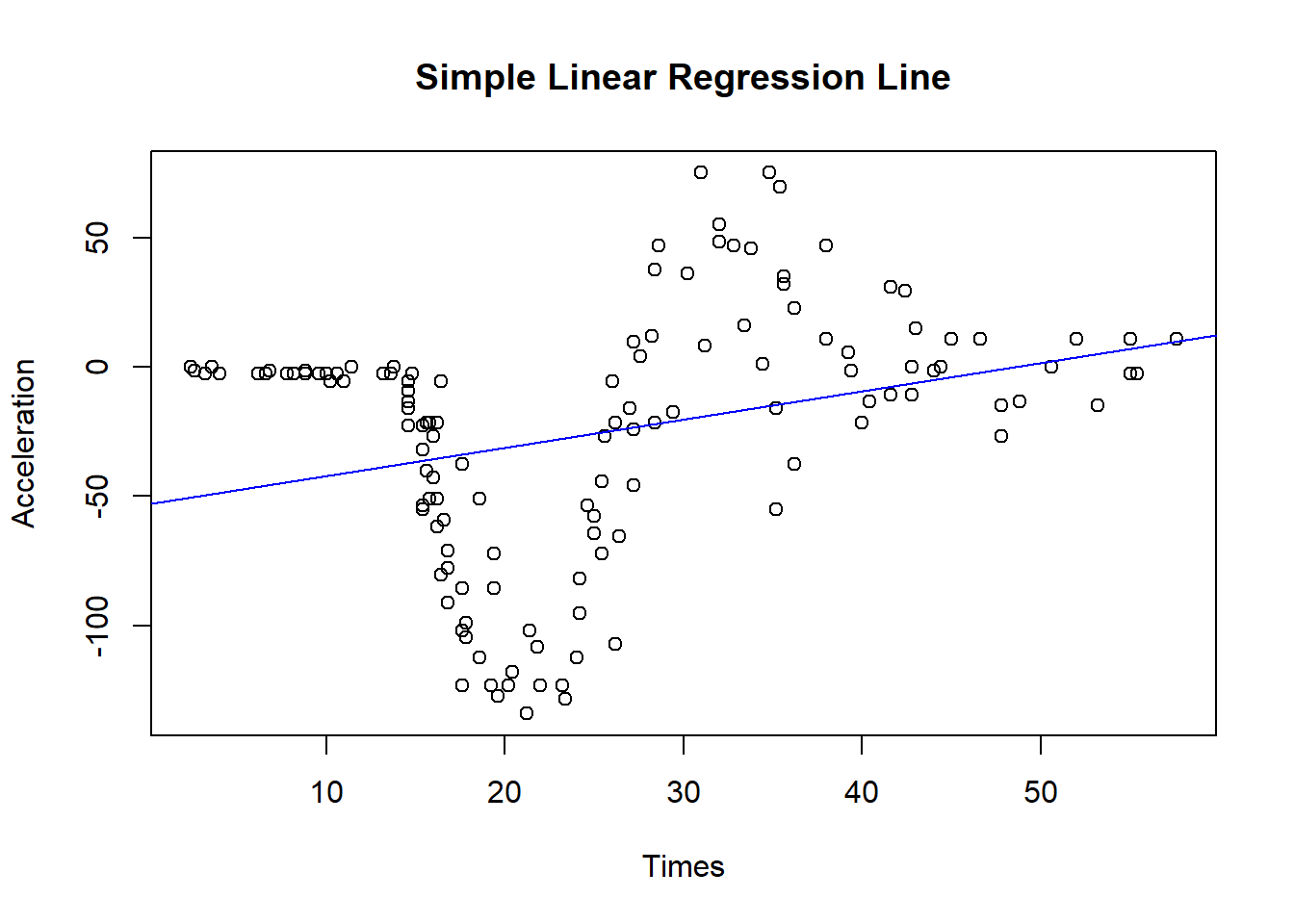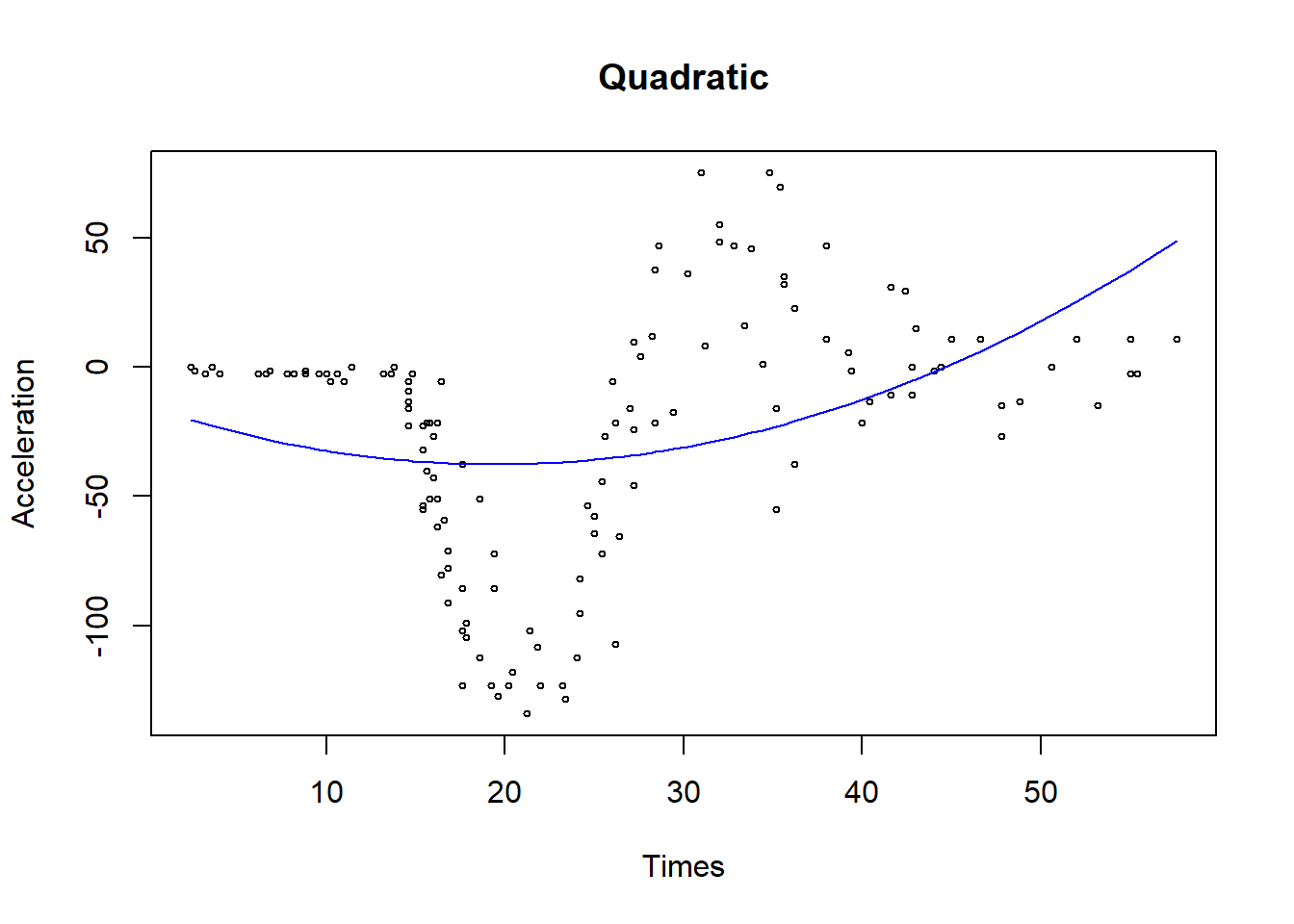# 1mcycle motorcycle accident dataset

`mcycle` is a data frame giving a series of measurements of head acceleration in a simulated motorcycle accident, used to test crash helmets. It is an univariate case. We are interested in the relationship between the times in milliseconds after impact and the acceleration.

``````library(MASS)
data('mcycle')
str(mcycle)
summary(mcycle)``````
``````# Rename the variables for ease of usage
Y <- mcycle\$accel
X <- mcycle\$times

#Scatterplot
plot(Y~X, xlab="time",ylab="Acceleration", main="Scatterplot of Acceleration against Time")``````# 2 Simple Linear Regression

We can simply assume a linear relationship and run a linear model between acceleration and time.

``````lm_mod <- lm(Y~X, data= mcycle)
summary(lm_mod)``````

Fitted Regression Line

But after we draw the fitted linear line on the scatterplot, we can clearly tell that the linear assumption seems violated.

``````plot(X, Y, xlab="Times", ylab="Acceleration", main="Simple Linear Regression Line")
abline(lm_mod, col="blue", lwd = 1)``````# 3 Polynomial Regression

If we assume there could be a polynomial relationship, we can try polynomial regression. The coefficients can be easily estimated using least squares linear regression because this is just a standard linear model with predictors \(x, x^2, x^3, \dots, x^d\).

We can conduct a quadratic regression as follows by simply adding `I(X^2)` in the formula argument:

``````quad_mod <- lm(Y~X+I(X^2), data=mcycle)
``````##
## Call:
## lm(formula = Y ~ X + I(X^2), data = mcycle)
##
## Residuals:
##     Min      1Q  Median      3Q     Max
## -96.527 -30.817   9.589  29.210 104.728
##
## Coefficients:
##              Estimate Std. Error t value Pr(>|t|)
## (Intercept) -15.22700   15.49471  -0.983  0.32757
## X            -2.30749    1.20439  -1.916  0.05757 .
## I(X^2)        0.05935    0.02038   2.912  0.00422 **
## ---
## Signif. codes:  0 '***' 0.001 '**' 0.01 '*' 0.05 '.' 0.1 ' ' 1
##
## Residual standard error: 45.06 on 130 degrees of freedom
## Multiple R-squared:  0.1437, Adjusted R-squared:  0.1306
## F-statistic: 10.91 on 2 and 130 DF,  p-value: 4.167e-05``````

It seems that the fitted line captures the curvature a little bit better than the linear regression.

``````plot(X ,Y ,xlab="Times", main = "Quadratic",ylab="Acceleration",cex=.5)Is this model superior to the simple linear regression model?

In order to answer this question, we can conduct the ANOVA test to compare the fits of these two models.

``anova(lm_mod,quad_mod)``

## 3.2 Fifth-degree Polynomial

We can also try higher order polynomial regress, for example a fifth-degree polynomial.

``````poly_mod <- lm(Y~poly(X,5,raw=T),data=mcycle)
summary(poly_mod)``````
``````##
## Call:
## lm(formula = Y ~ poly(X, 5, raw = T), data = mcycle)
##
## Residuals:
##     Min      1Q  Median      3Q     Max
## -77.271 -21.285   0.975  25.386  82.371
##
## Coefficients:
##                        Estimate Std. Error t value Pr(>|t|)
## (Intercept)          -1.059e+02  3.499e+01  -3.026    0.003 **
## poly(X, 5, raw = T)1  4.978e+01  1.036e+01   4.804 4.31e-06 ***
## poly(X, 5, raw = T)2 -6.359e+00  1.015e+00  -6.266 5.30e-09 ***
## poly(X, 5, raw = T)3  2.969e-01  4.253e-02   6.982 1.45e-10 ***
## poly(X, 5, raw = T)4 -5.742e-03  7.932e-04  -7.239 3.83e-11 ***
## poly(X, 5, raw = T)5  3.919e-05  5.406e-06   7.250 3.60e-11 ***
## ---
## Signif. codes:  0 '***' 0.001 '**' 0.01 '*' 0.05 '.' 0.1 ' ' 1
##
## Residual standard error: 33.9 on 127 degrees of freedom
## Multiple R-squared:  0.5264, Adjusted R-squared:  0.5078
## F-statistic: 28.24 on 5 and 127 DF,  p-value: < 2.2e-16``````

You can also assess the model performance.

``````#poly_mod_summary <- summary(poly_mod)
#(poly_mod_summary\$sigma)^2
#poly_mod_summary\$r.squared
``````plot(X ,Y ,xlab="Times", main = "Fifth-degree polynomial",ylab="Acceleration",cex=.5)